## 前言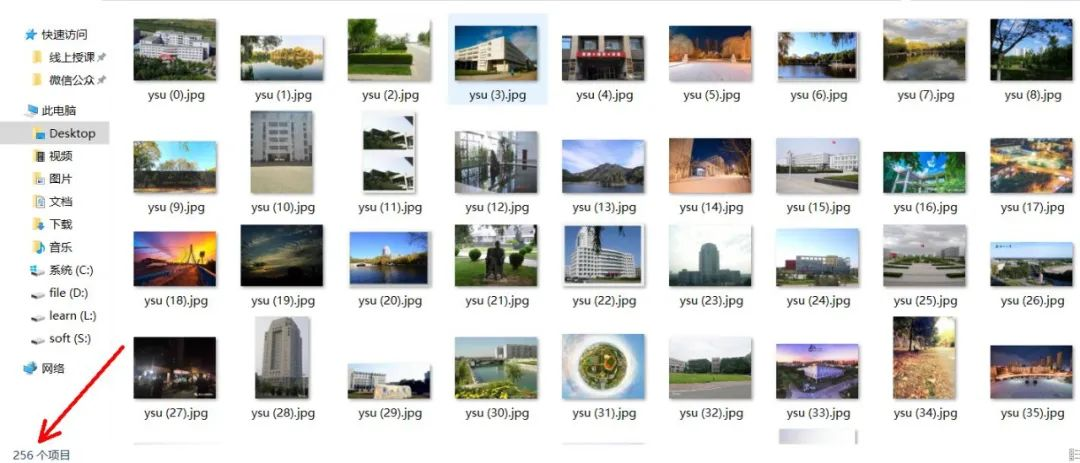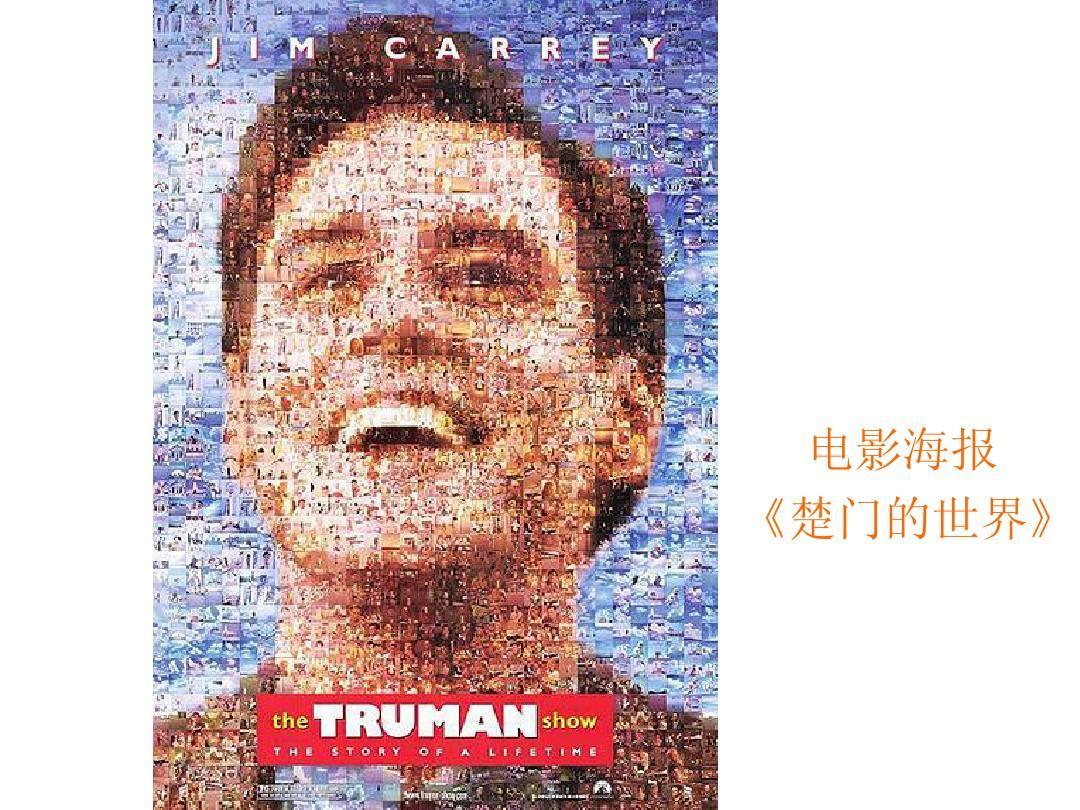### 综述实现思路

1：读取文件夹内的风景图片集，将每张剪裁到90*45大小并存入Mat容器内

2：将图片模板（彦的照片）扩大为1600*2700大小

3：计算图片集的直方图并将结果存到MatND容器内（直方图容器）

4：双重for循环以90*45的步长遍历图片模板计算各个区域的直方图，并将区域直方图与图片集的直方图进行比对，得到相似度最高的风景图片，将该风景图片替换模板对应区域

5：将4步得到的蒙太奇图与原模板图线性相加，得到更为逼真的效果

### 1：读取图片集并预处理

``````//【1】图片集的采集与处理
int Images_number = 256;//图片集中图片的数量
int step_x = 80; //将图片剪裁为80*45大小
int step_y = 45;

Mat srcImage;
vector<Mat> load_Images;//图片集容器
char filename;//存储图片名字
for (int i = 0; i < Images_number; i++) {
Mat dstImage;
sprintf_s(filename, "./风景图/ysu (%d).jpg", i);
cout << filename << endl;
srcImage = imread(filename); //Mat类图像
resize(srcImage, dstImage, Size(step_x, step_y), 0, 0, INTER_NEAREST);
load_Images.push_back(dstImage);
}
cout << "图片加载完毕" << endl;
``````

``````
``````

### 2：调整原模板图的尺寸大小

``````//【2】将原模板图扩大到合适尺寸
Mat originalImage, showImage;
originalImage = imread("彦.jpg");
imshow("彦", originalImage);
resize(originalImage, showImage, Size(1600, 2700));
``````

### 3：计算图片集直方图

``````//【3】计算图片集的直方图
int width = showImage.cols; //模板图的长宽
int height = showImage.rows;
Mat frame;
vector<MatND> hsit_list;//直方图容器
//计算直方图的参数准备
int bins = 128; //直条数
int hist_sizes[] = { bins,bins,bins }; //存放每个维度的直方图尺寸数组 //  均为256条宽度
float range[] = { 0,256 };  //每一维数组的取值范围  // 均为0-255高度
const float* ranges[] = { range,range,range };
int channels[] = { 0,1,2 };
//for循环计算图片集的直方图并存储
for (int i = 0; i < Images_number; i++) {
/*load_Images[i].copyTo(frame);*/
MatND hsit_RGB;
Mat frame;
load_Images[i].copyTo(frame);
calcHist(&frame, 1, channels, Mat(), hsit_RGB, 3, hist_sizes, ranges, true, false);
hsit_list.push_back(hsit_RGB);
}
``````

### 4：遍历彦模板图，计算每一小块的直方图并对比，替换

``````//【4】遍历，寻找最匹配的图片并替换
int number_order;
for (int x = 0; x < height; x = x + step_y) {
for (int y = 0; y < width; y = y + step_x) {
Mat roiImage = montageImage(Rect(y, x, step_x, step_y));//Rect(y, x, step_x, step_y)
//参数准备
MatND hsit_roi;
double match_ = 0.0;//匹配度
calcHist(&roiImage, 1, channels, Mat(), hsit_roi, 3, hist_sizes, ranges, true, false);
for (int i = 0; i < Images_number; i++) {
double match;
match = compareHist(hsit_roi, hsit_list[i], HISTCMP_CORREL);
if (match > match_) {//寻找对比对最高的
number_order = i;
match_ = match;
}
}
load_Images[number_order].copyTo(roiImage);
}
}
cout << "【4】遍历，寻找最匹配的图片并替换成功" << endl;
``````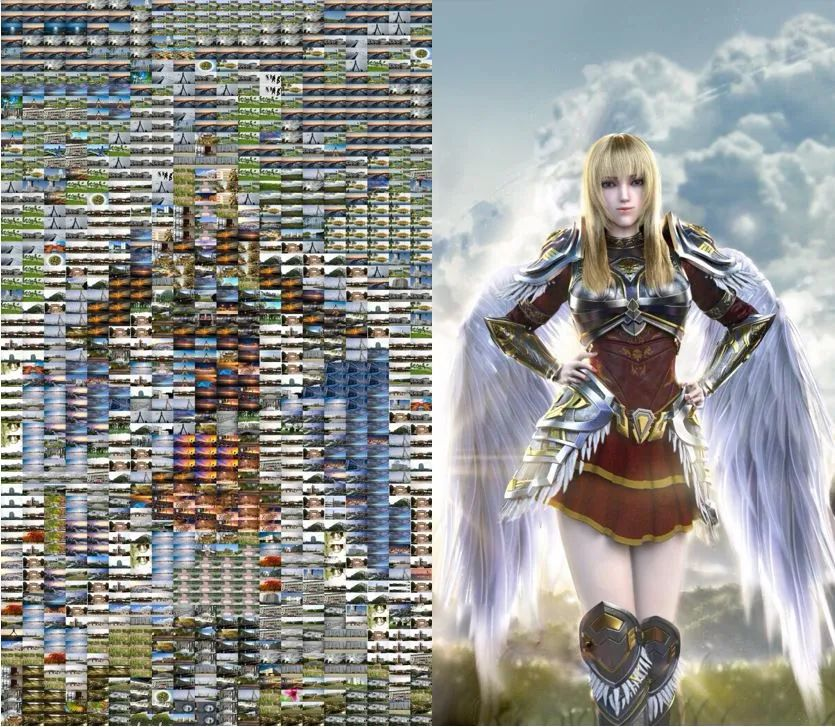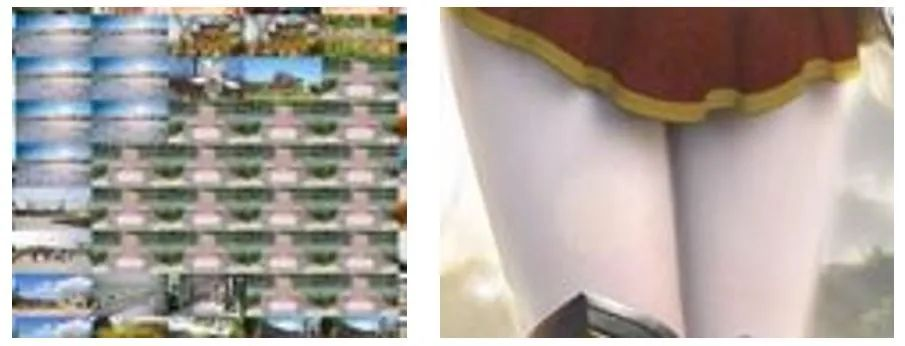### 5：将效果图与原图加和

``````Mat dstImage;
addWeighted(showImage, 0.4, montageImage, 0.6, 0, dstImage);
imwrite("结果图.jpg", dstImage);
imwrite("show.jpg", montageImage);
``````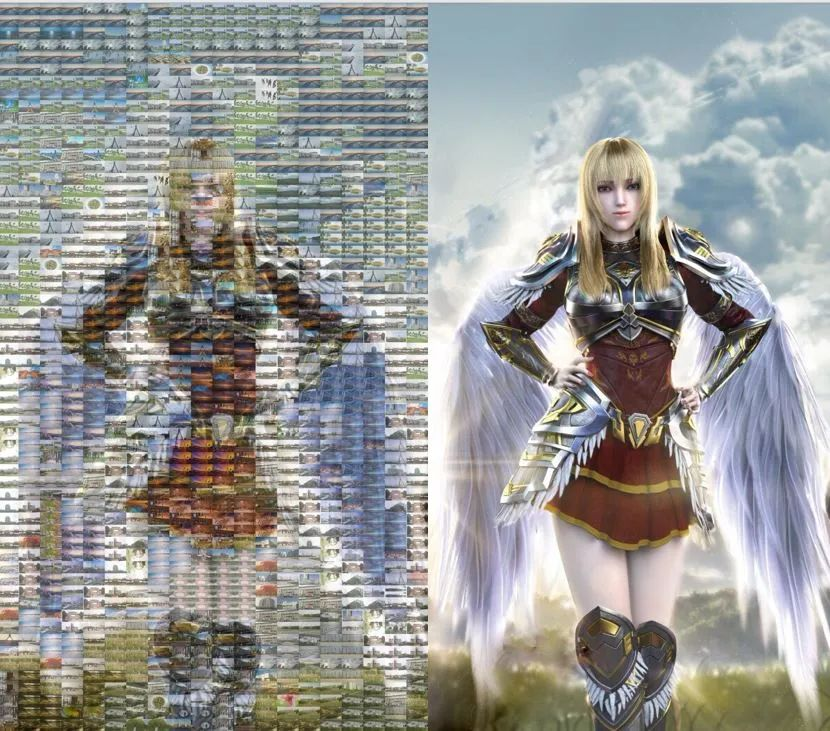### THE END

``````
``````